# Error 4806 while using CopyBuffer()257

Hi,

I would like to just get the value of a custom indicator in an Expert Advisor, here is my code :

```double   IndicatorBuffer[];
int      HandleIndicator = 0;

int OnInit()
{
HandleIndicator = iCustom(Symbol(),PERIOD_CURRENT,"MAindicator","EURUSD",PERIOD_CURRENT);

Print("iCustom HANDLE "+HandleIndicator+" ERR? "+GetLastError());

if(HandleIndicator > 0)

int CpyHandle = CopyBuffer(HandleIndicator,0,0,3,CorrelationBuffer);

Print("Cpy HANDLE "+CpyHandle+" ERR? "+GetLastError());
}
}```

Which give me :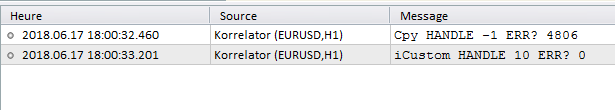Is it the correct way to get a value of a iCustom indicator ?

Why do I get this error ?27545

Get the handle in OnInit, the indicator hasn't yet run. Get the data in OnCalculate/OnTick after it has.257

whroeder1:
Get the handle in OnInit, the indicator hasn't yet run. Get the data in OnCalculate/OnTick after it has.

I tryed to put then to put the CopyBuffer in OnTick() as this code :

```double   IndicatorBuffer[];
int      HandleIndicator = 0;

int OnInit()
{
HandleIndicator = iCustom(Symbol(),PERIOD_CURRENT,"correlation","EURUSD",PERIOD_CURRENT);

Print("iCustom HANDLE "+HandleIndicator+" ERR? "+GetLastError());

return(INIT_SUCCEEDED);
}
void OnTick()
{
if(HandleIndicator > 0)
{
int CpyHandle = CopyBuffer(HandleIndicator,0,0,3,IndicatorBuffer);

Print("Cpy HANDLE "+CpyHandle+" ERR? "+GetLastError());
}

Print("First value "+IndicatorBuffer);
}```

But it still an error "Not all data of MA is calculated. Error 4806." :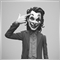34881

Kray:

I tryed to put then to put the CopyBuffer in OnTick() as this code :

But it still an error "Not all data of MA is calculated. Error 4806." :

Call the iCustom in OnTick()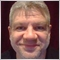43263

Call the iCustom in OnTick()
Don't do that, it's the best way to have problems.43263

Kray:

I tryed to put then to put the CopyBuffer in OnTick() as this code :

But it still an error "Not all data of MA is calculated. Error 4806." :

Don't check for error when there is no error.

What is this message "Not all data of MA...", it's not in the code you posted. Show you real code.257

Alain Verleyen:

Don't check for error when there is no error.

What is this message "Not all data of MA...", it's not in the code you posted. Show you real code.

This is exactly all the code I did in the Expert, this is the real code.

And I am calling the indicator "correlation" with iCustom, this is exactly this indicator : https://www.mql5.com/en/code/897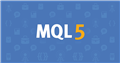Pearson correlation indicator
• 2012.05.30
• Mihail Lagutin
• www.mql5.com
Pearson correlation indicator shows the correlation between symbols. Input parameters: Symbol               - Name of the second symbol, used for calculation of correlation; Period                - Period; Price                  - Applied price; Min correlation  - Gradient color for weak...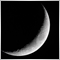11

Hi everyone,

I just start playing with mql5  by trying to create the MA cross custom indicator , after several tries I do have the same issue.

in my OnInit I launch my iMA handler

```int OnInit(){
MA1_hdl = iMA(Symbol(),Period(),MA1Period,0,MA1Mode,PRICE_CLOSE);
MA2_hdl = iMA(Symbol(),Period(),MA2Period,0,MA2Mode,PRICE_CLOSE);
//--- if the handle is not created
if( (MA1_hdl == INVALID_HANDLE) || (MA2_hdl == INVALID_HANDLE) ) {
//--- tell about the failure and output the error code
PrintFormat("Failed to create handle of the iMA indicator for the symbol %s/%s, error code %d",
Symbol(),
EnumToString(Period()),
GetLastError());
//--- the indicator is stopped early
return(INIT_FAILED);
}
}
```

and in OnCalculate I do the CopyBuffer of my iMA but it gives the same error :

-> Failed to copy data from the iMA indicator buffer   error code 4806

I have tried to insert a Sleep(5000) //5s  at the end of OnInit but it does NOT help.

any idea guys ?

/k43263

Karim Ouljihate:

Hi everyone,

I just start playing with mql5  by trying to create the MA cross custom indicator , after several tries I do have the same issue.

in my OnInit I launch my iMA handler

and in OnCalculate I do the CopyBuffer of my iMA but it gives the same error :

-> Failed to copy data from the iMA indicator buffer   error code 4806

I have tried to insert a Sleep(5000) //5s  at the end of OnInit but it does NOT help.

any idea guys ?

/k

Show all the relevant code if you need help.11

Hello everyone.

I also got Error 4806 while creating a multi-timeframe indicator, so I will write about how to deal with it.

The environment at that time was _Period = PERIOD_D1, MTF_Period = PERIOD_W1.

Below is the error and the corresponding code. Please note that only points.

2019.10.12 00: 00: 00.182 MADS (GBPJPY, D1) Time: 1993.04.19 00:00

2019.10.12 00: 00: 00.188 MADS (GBPJPY, D1) Getting EMA failed! Error4806

``` int OnInit ()
{
ExtFastMaHandle= iMA ( NULL , 0 ,f_ma_period, 0 , MODE_EMA , PRICE_CLOSE );
ExtMiddleMaHandle= iMA ( NULL , 0 ,m_ma_period, 0 , MODE_EMA , PRICE_CLOSE );
ExtSlowMaHandle= iMA ( NULL , 0 ,s_ma_period, 0 , MODE_EMA , PRICE_CLOSE );
ExtFastMaHandle2= iMA ( NULL ,mtf_timeframe,f_ma_period, 0 , MODE_EMA , PRICE_CLOSE );
ExtMiddleMaHandle2= iMA ( NULL ,mtf_timeframe,m_ma_period, 0 , MODE_EMA , PRICE_CLOSE );
ExtSlowMaHandle2= iMA ( NULL ,mtf_timeframe,s_ma_period, 0 , MODE_EMA , PRICE_CLOSE );

return ( INIT_SUCCEEDED );
}

//+------------------------------------------------------------------+
//| Universal Moving Average                                         |
//+------------------------------------------------------------------+
int OnCalculate ( const int rates_total,
const int prev_calculated,
const datetime &time[],
const double &open[],
const double &high[],
const double &low[],
const double &close[],
const long &tick_volume[],
const long &volume[],
{
//--- check for data
if (rates_total==prev_calculated)
{
return (rates_total);
}

if (!_CHK_BarCal(ExtFastMaHandle,rates_total))
return ( 0 );
if (!_CHK_BarCal(ExtMiddleMaHandle,rates_total))
return ( 0 );
if (!_CHK_BarCal(ExtSlowMaHandle,rates_total))
return ( 0 );

//--- we can copy not all data
int to_copy;
if (prev_calculated>rates_total || prev_calculated< 0 )
to_copy=rates_total;
else
{
to_copy=rates_total-prev_calculated;
if (prev_calculated> 0 )
to_copy++;
}

if (!_CopyBuffer(ExtFastMaHandle,to_copy,ExtFastMaBuffer))
return ( 0 );
if (!_CopyBuffer(ExtMiddleMaHandle,to_copy,ExtMiddleMaBuffer))
return ( 0 );
if (!_CopyBuffer(ExtSlowMaHandle,to_copy,ExtSlowMaBuffer))
return ( 0 );

int mtf_bars = Bars ( NULL , mtf_timeframe);
if (mtf_bars < s_ma_period)
return ( 0 );

if (!_CHK_BarCal(ExtFastMaHandle2,mtf_bars))
return ( 0 );
if (!_CHK_BarCal(ExtMiddleMaHandle2,mtf_bars))
return ( 0 );
if (!_CHK_BarCal(ExtSlowMaHandle2,mtf_bars))
return ( 0 );

//--- set limit for which bars need to be (re)calculated
int limit;
if (prev_calculated== 0 )
limit= 0 ;
else
limit=prev_calculated- 1 ;

for ( int i=limit; i<rates_total && ! IsStopped (); i++)
{
if (!_CopyBuffer(ExtFastMaHandle2,time,ExtFastMaBuffer2,i))
return ( 0 );
if (!_CopyBuffer(ExtMiddleMaHandle2,time,ExtMiddleMaBuffer2,i))
return ( 0 );
if (!_CopyBuffer(ExtSlowMaHandle2,time,ExtSlowMaBuffer2,i))
return ( 0 );

}

//Print("Time:",TimeToString(time));

return (rates_total);
}

//+------------------------------------------------------------------+
//|                                                                  |
//+------------------------------------------------------------------+
bool _CHK_BarCal( int handle, int bars)
{
int cal = BarsCalculated (handle);
if (cal < bars)
{
//Print("Not all data is calculated (",cal,"bars ). Error",GetLastError());
return ( false );
}
return ( true );
}

//+------------------------------------------------------------------+
//|                                                                  |
//+------------------------------------------------------------------+
bool _CopyBuffer( int handle, int num, double &buffer[])
{
if ( IsStopped ())
return ( false ); //Checking for stop flag
if ( CopyBuffer (handle, 0 , 0 ,num,buffer)<= 0 )
{
Print ( "Getting EMA is failed! Error" , GetLastError ());
return ( false );
}
return ( true );
}
bool _CopyBuffer( int handle, const datetime &time[], double &buf[], int i)
{
double result[ 1 ];
if ( CopyBuffer (handle, 0 ,time[i], 1 ,result)> 0 )
{
buf[i] = result[ 0 ];
return ( true );
}
else
{
Print ( "Time:" , TimeToString (time[i]));
Print ( "Getting EMA failed! Error" , GetLastError ());
return ( false );
}
}
```

The error is in the second _CopyBuffer ().

And this is the starting point with _Period = PERIOD_W1.

2019.10.12 00: 47: 40.231 MADS (GBPJPY, W1) Time: 1993.04.18 00:00

``` int OnCalculate ( const int rates_total,
const int prev_calculated,
const datetime &time[],
const double &open[],
const double &high[],
const double &low[],
const double &close[],
const long &tick_volume[],
const long &volume[],
{
//--- check for data
if (rates_total==prev_calculated)
{
return (rates_total);
}

Print ( "Time:" , TimeToString (time[ 0 ]));

return (rates_total);
}

```

From here, I guess, I think this is similar to the following problem.

```   int n;
datetime date1 = D'2016.09.02 23:55' ; // Friday
datetime date2 = D'2016.09.05 00:00' ; // Monday
datetime date3 = D'2016.09.08 00:00' ; // Thursday
//---
n= Bars ( _Symbol , PERIOD_H1 , D'2016.09.02 02:05' , D'2016.09.02 10:55' );
Print ( "Number of bars: " ,n); // Output: "Number of bars: 8", H2 bar is considered in the calculation, while H11 one is not
n= Bars ( _Symbol , PERIOD_D1 ,date1,date2);
Print ( "Number of bars: " ,n); // Output: "Number of bars: 1", since an open time of a single D1 (Monday) bar falls within the interval
n= Bars ( _Symbol , PERIOD_W1 ,date2,date3);
Print ( "Number of bars: " ,n); // Output: "Number of bars: 0", since not a single W1 bar open time falls within the specified interval ```

Therefore, in this error, we think that time [i] needs to be converted to the upper leg, or several reading start legs need to be done first.

I changed the following part in order to make the reading start point first.

``` //--- set limit for which bars need to be (re)calculated
int limit;
if (prev_calculated== 0 )
limit= 10 ; // limit=0;  <<<<<<<<<<<<<<<<<<<<<< Change !!!
else
limit=prev_calculated- 1 ;

for ( int i=limit; i<rates_total && ! IsStopped (); i++)
{
if (!_CopyBuffer(ExtFastMaHandle2,time,ExtFastMaBuffer2,i))
return ( 0 );
```

I think that there is no problem as long as there is a necessary width for this value.

I think your problem (Error 4806) is fundamentally the same, so I hope you find my example helpful.Documentation on MQL5: Timeseries and Indicators Access / Bars
• www.mql5.com
If the start_time and stop_time parameters are defined, the function returns the number of bars in the specified time interval, otherwise it returns the total number of bars. If data for the timeseries with specified parameters are not formed in the terminal by the time of the Bars() function call, or data of the timeseries are not synchronized...7

check this:
```if (BarsCalculated(handlerMtf) < 0)
{